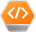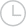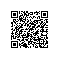# 应该返回false的用户输入返回true-问答-阿里云开发者社区-阿里云

## 应该返回false的用户输入返回true被纵养的懒猫2019-10-09 17:50:08336

true==false？什么时候应该使用它，不应该使用它？有什么不同？

== 测试引用是否相等（它们是否是同一对象）。

.equals() 测试值是否相等（在逻辑上是否为“相等”）。

Objects.equals（）null在调用之前进行检查，.equals()因此您不必（在JDK7起可用，在Guava中也可用）。

String.contentEquals（）将的内容String与任何内容进行比较CharSequence（从Java 1.5开始可用）。

// These two have the same value new String("test").equals("test") // --> true

// ... but they are not the same object new String("test") == "test" // --> false

// ... neither are these new String("test") == new String("test") // --> false

// ... but these are because literals are interned by // the compiler and thus refer to the same object "test" == "test" // --> true

// ... string literals are concatenated by the compiler // and the results are interned. "test" == "te" + "st" // --> true

// ... but you should really just call Objects.equals() Objects.equals("test", new String("test")) // --> true Objects.equals(null, "test") // --> false Objects.equals(null, null) // --> true 您几乎总是想使用Objects.equals()。在极少数情况下，您知道要处理实习生字符串，可以使用==。

==测试对象引用，.equals()测试字符串值。

String fooString1 = new String("foo"); String fooString2 = new String("foo");

// Evaluates to false fooString1 == fooString2;

// Evaluates to true fooString1.equals(fooString2);

// Evaluates to true, because Java uses the same object "bar" == "bar"; 但要注意空值！

==可以null很好地处理字符串，但是.equals()从空字符串调用将导致异常：

String nullString1 = null; String nullString2 = null;

// Evaluates to true System.out.print(nullString1 == nullString2);

// Throws a NullPointerException System.out.print(nullString1.equals(nullString2)); 因此，如果您知道该值fooString1可能为空，请告诉读者

System.out.print(fooString1 != null && fooString1.equals("bar")); 以下内容比较简短，但检查空值（来自Java 7）不太明显：

System.out.print(Objects.equals(fooString1, "bar"));

@JonCoombs Java支持子类化和创建自己的方法。但是由于某些原因，很少有类被标记为final，String是其中之一，因此我们无法扩展。我们可以在其中创建其他类并制作实用程序类，该类以两个字符串作为参数，并在那里实现我们的逻辑。同样，对于null检查其他一些库（例如spring和apache），也可以使用该库

== 比较对象引用。

.equals() 比较字符串值。

String a="Test"; String b="Test"; if(a==b) ===> true 这是因为当您创建任何String文字时，JVM首先在String池中搜索该文字，并且如果找到匹配项，则将对新String赋予相同的引用。因此，我们得到：

（a == b）===>是

``````                   String Pool
b -----------------> "test" <-----------------a
``````

String a="test"; String b=new String("test"); if (a==b) ===> false 在这种情况下，new String("test")将在堆上创建语句new String，并将对该引用进行引用b，因此b将在堆上（而不是在String池中）为其提供引用。

if（a == b）===>否。

``````            String Pool
"test" <-------------------- a

Heap
"test" <-------------------- b
``````

String a="Test"; String b="Test"; if(a.equals(b)) ===> true

String a="test"; String b=new String("test"); if(a.equals(b)) ===> true 因此使用.equals()总是更好。

.equals（）比较两个实例，但是实现了equals来比较它们。那可能比较或不比较toString的输出。

@Jacob对象类.equals()方法比较实例（引用/地址），其中String类.equals()方法被覆盖以比较内容（字符）

Java中的字符串是不可变的。这意味着每当您尝试更改/修改字符串时，您都会获得一个新实例。您不能更改原始字符串。这样做是为了可以缓存这些字符串实例。典型的程序包含许多字符串引用，对这些实例进行缓存可以减少内存占用并提高程序性能。

Java还讨论了可以在字符串上使用以使其成为高速缓存一部分的函数intern（），因此"MyString" == new String("MyString").intern()将返回true。

String a = new String("foo"); String b = new String("foo"); System.out.println(a == b); // prints false System.out.println(a.equals(b)); // prints true 确保您了解原因。这是因为==比较只比较引用；该equals()方法对内容进行逐字符比较。

==表示您的两个字符串引用是完全相同的对象。您可能已经听说过这种情况，因为Java保留了某种文字表（它确实这样做了），但并非总是如此。某些字符串以不同的方式加载，它们是由其他字符串等构成的，因此，您绝不能假定两个相同的字符串存储在同一位置。

115

Java有一个String池，Java在该池下管理String对象的内存分配。请参见Java中的字符串池

equals 实际上是Object类的方法，但是它被重写为String类，并给出了一个新的定义来比较对象的内容。

Example: stringObjectOne.equals(stringObjectTwo); 但是请注意，它尊重String的大小写。如果要区分大小写，则必须使用String类的equalsIgnoreCase方法。

String one = "HELLO"; String two = "HELLO"; String three = new String("HELLO"); String four = "hello";

one == two; // TRUE one == three; // FALSE one == four; // FALSE

one.equals(two); // TRUE one.equals(three); // TRUE one.equals(four); // FALSE one.equalsIgnoreCase(four); // TRUE 分享改善这个答案 18年2月8日于19:19编辑 社区维基 4转，3用户80％ Saurabh Agarwal 6 我看到这是一个大问题的较晚答案。我可以问一下现有答案中没有提到的内容吗？ – 他添加了@Mysticial equalsIgnoreCase，这可能对新手有帮助。

// ... but they are not the same object new String("test") == "test" ==> false 如果您实习非字面性的字符串相等是 true

new String("test").intern() == "test" ==> true

==比较Java中的对象引用，对象也不例外String。

.equals()比较类中的数据（假设函数已实现）。 ==比较指针位置（对象在内存中的位置）。

==如果两个对象（不讲主旨）都指向SAME对象实例，则返回true。 .equals()如果两个对象包含的数据与Java中的相同，则返回trueequals()==

==执行引用相等性检查，检查这两个对象（在这种情况下为字符串）是否引用内存中的同一对象。

Obviously == is faster-实际上.equals(String)先执行==其他检查，然后说速度差不多。 public boolean equals(Object anObject) { if (this == anObject) { return true; } ...

if (string1.equals(string2)) 此String equals方法查看两个Java字符串，如果它们包含完全相同的字符串，则认为它们相等。

String string1 = "foo"; String string2 = "FOO";

if (string1.equals(string2)) { // this line will not print because the // java string equals method returns false: System.out.println("The two strings are the same.") } 但是，当两个字符串包含完全相同的字符串时，equals方法将返回true，如以下示例所示：

String string1 = "foo"; String string2 = "foo";

// test for equality with the java string equals method if (string1.equals(string2)) { // this line WILL print System.out.println("The two strings are the same.") } 选项2：使用equalsIgnoreCase方法进行字符串比较

String string1 = "foo"; String string2 = "FOO";

// java string compare while ignoring case if (string1.equalsIgnoreCase(string2)) { // this line WILL print System.out.println("Ignoring case, the two strings are the same.") } 选项3：使用compareTo方法比较Java字符串

String string1 = "foo bar"; String string2 = "foo bar";

// java string compare example if (string1.compareTo(string2) == 0) { // this line WILL print System.out.println("The two strings are the same.") } 当我写有关Java中的相等性的概念时，必须注意Java语言在基本Java Object类中包含equals方法。每当创建自己的对象时，只要想提供一种方法来查看对象的两个实例是否相等，就应该在类中重写（并实现）此equals方法（以Java语言提供的相同方式） String equals方法中的这种相等/比较行为）。

public float simpleSimilarity(String u, String v) { String[] a = u.split(" "); String[] b = v.split(" ");

``````long correct = 0;
int minLen = Math.min(a.length, b.length);

for (int i = 0; i < minLen; i++) {
String aa = a[i];
String bb = b[i];
int minWordLength = Math.min(aa.length(), bb.length());

for (int j = 0; j < minWordLength; j++) {
if (aa.charAt(j) == bb.charAt(j)) {
correct++;
}
}
}

return (float) (((double) correct) / Math.max(u.length(), v.length()));
``````

} 测试：

String a = "This is the first string.";

String b = "this is not 1st string!";

// for exact string comparison, use .equals

boolean exact = a.equals(b);

// For similarity check, there are libraries for this // Here I'll try a simple example I wrote

float similarity = simple_similarity(a,b);

String s1 = "Stack Overflow"; String s2 = "Stack Overflow"; s1 == s2; //true s1.equals(s2); //true 原因：不带空值创建的字符串文字存储在堆的permgen区域的字符串池中。因此s1和s2都指向池中的同一对象。

String s1 = new String("Stack Overflow"); String s2 = new String("Stack Overflow"); s1 == s2; //false s1.equals(s2); //true 原因：如果使用new关键字创建String对象，则会在堆上为其分配单独的空间。

==比较对象的参考值，而类中equals()存在的方法java.lang.String将String对象的内容（与另一个对象）进行比较。

@JacobSchoen：由于GrepCode关闭，上述链接不再起作用。这是equals实现的替代方法：[内联链接]（zgrepcode.com/java/openjdk/10.0.2/java.base/java/lang/…） – Amandeep Singh 1月27日在6:16

str1 = new String("abc"); str2 = new String("abc"); 如果您使用 equals()

System.out.println((str1.equals(str2))?"TRUE":"FALSE"); 您将获得与TRUE使用一样的输出==。

System.out.println((str1==str2) ? "TRUE" : "FALSE"); 现在，您将获得FALSEas输出，因为str1和str2都指向两个不同的对象，即使它们都共享相同的字符串内容。这是因为new String()每次都会创建一个新对象。

String s1 = new String("abc"); String s2 = new String("abc"); System.out.println(s1 == s2); // It prints false (reference comparison) System.out.println(s1.equals(s2)); // It prints true (content comparison) 分享改善这个答案 18年2月8日于19:20编辑 社区维基 3转，2个用户， sham.y为54％

.equals()由于Object包含一个.equals()返回布尔值的方法，因此保证所有对象都具有一个方法。如果需要进一步定义，则子类的工作就是覆盖此方法。没有它（即使用==），仅检查两个对象之间的内存地址是否相等。字符串重写此.equals()方法，并且不使用内存地址，而是返回字符级别的字符串比较以确保相等。

“一旦创建了字符串，它将永远存储在程序中的相同地址上” -这是绝对错误的。只有编译时常量字符串表达式（可能涉及final String变量）和程序显式实习的字符串才存储在所谓的“集总池”中。String一旦没有其他活动对象像其他任何类型的对象一样，所有其他对象也将进行垃圾回收。同样，虽然整个实习机制必须具备不变性，但与此无关。 –泰德·霍普 （ Ted Hopp）2014年4月10日在18:13 字符串比较是通过equals或equalsIgnoreCase方法完成的，该方法实际上是比较字符串的内容。但是==符号只是检查参考值。对于这种情况，来自字符串池的字符串文字可以正常工作。字符串s1 =新的String（“ a”）; 字符串s2 =新的String（“ a”）; 在这种情况下，s1 == s2为假，但s1.equals（s2）为真。 您也可以使用该compareTo()方法比较两个字符串。如果compareTo结果为0，则两个字符串相等，否则比较的字符串不相等。

Java String类实际上覆盖了Object类中默认的equals（）实现，并且覆盖了该方法，以便它仅检查字符串的值，而不检查它们在内存中的位置。这意味着，如果调用equals（）方法比较2个String对象，则只要实际字符序列相等，就认为这两个对象相等。取消 提交回答使用钉钉扫一扫加入圈子
+ 订阅